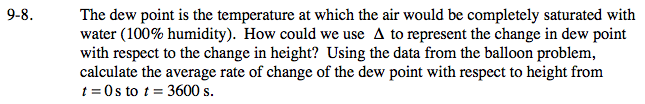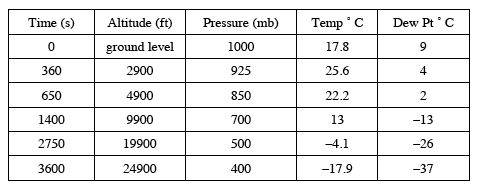### Home > PCT > Chapter Ch9 > Lesson 9.1.1 > Problem9-8

9-8.

The dew point is the temperature at which the air would be completely saturated with water (100% humidity). How could we use Δ to represent the change in dew point with respect to the change in height? Using the data from the balloon problem, calculate the average rate of change of the dew point with respect to height from t = 0 s to t = 3600 s. Homework Help ✎$\frac{\Delta\text{ dewpoint}}{\Delta\text{ height}} = \frac{-37 - 9}{24900 - 0}$